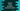# Python program to check if a number is abundant/excessive or not## Python program to check if a number is an abundant/ excessive number or not :

In this tutorial, we will learn how to find if a number is an abundant/excessive number or not using python. A number is called an abundant number or excessive number_ if the sum of all of its proper divisors is greater than the number itself. A proper divisor of a number is any divisor of that number other than the number itself. For example, _6 has proper divisor 1,2 and 3. All prime numbers have only one proper divisor i.e. 1 and other numbers have at least two proper divisors.

For an abundant or excessive number, the sum of its all proper divisors should be greater than the number itself. 12 is an abundant number. Because its proper divisors are 1,2,3,4 and 6__. The sum of all proper divisors is 1+2+3+4+6 = 16 which is greater than 12. Similarly, 54, 88, 100,102,112 etc. all are abundant number.

In our program, we will learn how to check if a number is abundant or not using a user provided value.The user will enter one number and the program will check if it is abundant or not and it will print out the result accordingly.

### Python program :

``````#1
input_no = int(input("Enter a number : "))
total = 0

#2
is_abundant = 0

#3
for i in range(1,input_no):
#4
if(input_no % i == 0):
#5
total = total + i
if(total > input_no):
is_abundant = 1
break

#6
if((total > input_no) or (is_abundant ==1)):
print("It is an abundant number.")
else :
print("It is not an abundant number.")``````

### Explanation :

The commented numbers in the above program denote the step-number below :

1. Ask the user to enter a number and save it in the inputno_ variable. Also, initialize one number total to store the sum of all proper divisors of the number. We are using_ input()_ to read the user input number. input() will read it as a string. So, we are wrapping it with int() to convert the value to an integer.
2. isabundant_ is a flag to detect if the number is abundant or not. 1 means it is an abundant number. 0 means not.
3. Start one for loop to run from_ 1 to inputno -1. We are using this loop to find out all the proper divisors for the given number.
4. Check for each number, if it is a proper divisor or not. no%i returns the remainder of_ no/i. So, we are checking if the remainder is _0 or not. If yes, it is a proper divisor.
5. If it is a proper divisor, add this number to the total sum variable total. Also, check if the total is more than the user-input no. or not. If yes, it is an abundant number. So, we don’t need to check any further. Set the value to isabundant_ as 1 and break from the loop.
6. Finally, check if the total sum total is more than inputnoor if _isabundantis equal to _1 or not. We are checking both cases because if the for loop runs for all numbers, isabundant_ will be 0. Else, if we found the total more than inputnoin the middle of the loop, _isabundant_ will be 1. Print out the message to the user if it is an abundant number or not.

### Conclusion :

In this tutorial, we have learned how to find out if a number is abundant or not using Python. Try to run the above example program and drop one comment below if you have any queries.